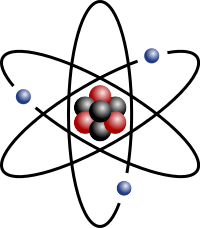## Average Atomic Mass

#### Learning Objective

• Calculate the average atomic mass of an element given its isotopes and their natural abundance

#### Key Points

• An element can have differing numbers of neutrons in its nucleus, but it always has the same number of protons. The versions of an element with different neutrons have different masses and are called isotopes.
• The average atomic mass for an element is calculated by summing the masses of the element’s isotopes, each multiplied by its natural abundance on Earth.
• When doing any mass calculations involving elements or compounds, always use average atomic mass, which can be found on the periodic table.

#### Terms

• natural abundanceThe abundance of a particular isotope naturally found on the planet.
• average atomic massThe mass calculated by summing the masses of an element’s isotopes, each multiplied by its natural abundance on Earth.
• mass numberThe total number of protons and neutrons in an atomic nucleus.

The atomic number of an element defines the element’s identity and signifies the number of protons in the nucleus of one atom. For example, the element hydrogen (the lightest element) will always have one proton in its nucleus. The element helium will always have two protons in its nucleus.

## Isotopes

Atoms of the same element can, however, have differing numbers of neutrons in their nucleus. For example, stable helium atoms exist that contain either one or two neutrons, but both atoms have two protons. These different types of helium atoms have different masses (3 or 4 atomic mass units), and they are called isotopes. For any given isotope, the sum of the numbers of protons and neutrons in the nucleus is called the mass number. This is because each proton and each neutron weigh one atomic mass unit (amu). By adding together the number of protons and neutrons and multiplying by 1 amu, you can calculate the mass of the atom. All elements exist as a collection of isotopes. The word ‘isotope’ comes from the Greek ‘isos’ (meaning ‘same’) and ‘topes’ (meaning ‘place’) because the elements can occupy the same place on the periodic table while being different in subatomic construction.Lithium AtomStylized lithium-7 atom: 3 protons (red), 4 neutrons (blue), and 3 electrons (black). (Lithium also has another, rarer isotope with only 2 neutrons. )

## Calculating Average Atomic Mass

The average atomic mass of an element is the sum of the masses of its isotopes, each multiplied by its natural abundance (the decimal associated with percent of atoms of that element that are of a given isotope).

Average atomic mass = f1M1 + f2M2 + … + fnMn where f is the fraction representing the natural abundance of the isotope and M is the mass number (weight) of the isotope.

The average atomic mass of an element can be found on the periodic table, typically under the elemental symbol. When data are available regarding the natural abundance of various isotopes of an element, it is simple to calculate the average atomic mass.

• For helium, there is approximately one isotope of Helium-3 for every million isotopes of Helium-4; therefore, the average atomic mass is very close to 4 amu (4.002602 amu).
• Chlorine consists of two major isotopes, one with 18 neutrons (75.77 percent of natural chlorine atoms) and one with 20 neutrons (24.23 percent of natural chlorine atoms). The atomic number of chlorine is 17 (it has 17 protons in its nucleus).

To calculate the average mass, first convert the percentages into fractions (divide them by 100). Then, calculate the mass numbers. The chlorine isotope with 18 neutrons has an abundance of 0.7577 and a mass number of 35 amu. To calculate the average atomic mass, multiply the fraction by the mass number for each isotope, then add them together.

Average atomic mass of chlorine = (0.7577 $\cdot$ 35 amu) + (0.2423 $\cdot$ 37 amu) = 35.48 amu

Another example is to calculate the atomic mass of boron (B), which has two isotopes: B-10 with 19.9% natural abundance, and B-11 with 80.1% abundance. Therefore,

Average atomic mass of boron = (0.199
$\cdot$

10 amu) + (0.801
$\cdot$

11 amu) = 10.80 amu

Whenever we do mass calculations involving elements or compounds (combinations of elements), we always use average atomic masses.